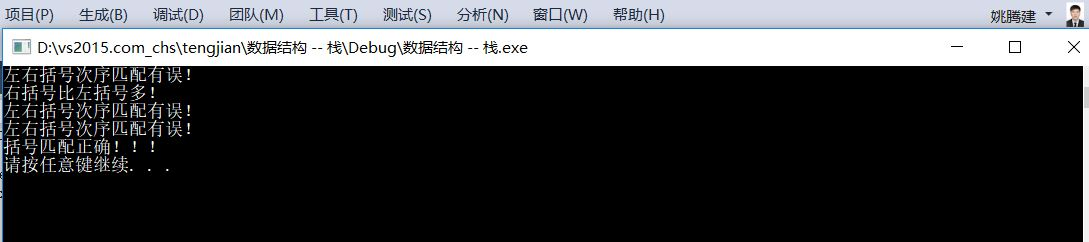括号匹配数据结构_数据结构实验之栈与队列四:括号匹配 测试数据 - CSDN
• 问题： 输入一个带有 '(' 、')'的字符串，编写程序判断左右括号个数是否匹配。 输入：（4+3）*5/（（34+2）-64） 思路： | 若有左括号压入栈中 输入字符串--->遍历各字符---> | ...
问题：

输入一个带有 '(' 、')'的字符串，编写程序判断左右括号个数是否匹配。

输入：（4+3）*5/（（34+2）-64）

思路：

|  若有左括号压入栈中

输入字符串--->遍历各字符--->   |                                                                            --->判断栈是否为空--->空--->匹配

|  若有右括号进入判断--->栈为空--->括号不匹配                                --->不空--->不匹配

--->栈不空--->将栈顶弹出

c++代码：

#include<stack>
#include<string>
#include<iostream>

using namespace std;
bool matchParenthesis(const string& s)
{
stack<char> let;
string::size_type len = s.length();
for(string::size_type i=0;i<len;i++)
{
char a = s.at(i);
if(a=='(')
let.push(a);
else if(a==')')
{
if(let.empty())
{
cout<<"false"<<endl;
return false;
}
let.pop();
}
}
if(let.empty())
{
cout<<"true"<<endl;
return true;
}
else
{
cout<<"false"<<endl;
return false;
}
}
int main(void)
{
string s;
cin>>s;
matchParenthesis(s);
}


展开全文括号匹配
• 数据结构括号匹配问题的解决
数据结构之括号匹配问题的解决参考文章： （1）数据结构之括号匹配问题的解决 （2）https://www.cnblogs.com/yjh123/p/5997833.html 备忘一下。
展开全文数据结构
• Description 输入一串字符串，编写算法判断字符串中的括号是否匹配，如果匹配，输出1，否则输出0。 注： 只考虑半角括号：( ) { } [ ]，不考虑全角括号：（ ） 【 ...字符串中的括号匹配。 {323[ab]()(123} 字...
个人博客网站：https://www.liuzhi.org.cn/

Description

输入一串字符串，编写算法判断字符串中的括号是否匹配，如果匹配，输出1，否则输出0。

注： 只考虑半角括号：( ) { } [ ]，不考虑全角括号：（ ） 【 】

例如：{ab123[(3*6-(4+3)) {223}hhh}

字符串中的括号匹配。

{323[ab]()(123}

字符串中的括号不匹配。

提示：利用栈实现。

Input

输入可以包含各种括号、数字、字母等符号的字符串。

Output

括号匹配输出1，否则输出0。

Sample Input

sample 1:
{ab123[(3*6-(4+3)){223}hhh}

sample 2:
{323[ab]()123}

Sample Output

sample1:
0
sample2:
1

#include <stdio.h>
#include <stdlib.h>
#include <string.h>
#define FALSE 0
#define TRUE 1
int  match(char a,char b)
{
if(a=='('&&b==')')
return TRUE;
if(a=='['&&b==']')
return TRUE;
if(a=='{'&&b=='}')
return TRUE;
return FALSE;
}
int Bice(char* s)
{
int i,n=strlen(s),top=-1;
char st[n];
for(i=0;i<n;i++)
{
if(s[i]==')'||s[i]==']'||s[i]=='}')
{
if(top>=0&&match(st[top],s[i]))
top--;
else
return FALSE;
}
else if(s[i]=='('||s[i]=='['||s[i]=='{')
st[++top]=s[i];
}
if(top==-1)
return TRUE;
return FALSE;
}
int main(){
char array;
gets(array);
int i;
i=Bice(array);
if(i==1){
printf("1");
}
else{
printf("0");
}
return 0;
}




展开全文• 给定一个字符串，其中的字符只包含三种括号：花括号{ }、中括号[ ]、圆...括号匹配要求括号必须以正确的顺序配对，如“{ [ ] ( ) }”或 “[ ( { } [ ] ) ]” 等为正确的格式，而“[ ( ] )”或“{ [ ( ) }”或“( { ...
       给定一个字符串，其中的字符只包含三种括号：花括号{ }、中括号[ ]、圆括号( )，即它仅由 “( ) [ ] { }” 这六个字符组成。设计算法，判断该字符串是否有效，即字符串中括号是否匹配。括号匹配要求括号必须以正确的顺序配对，如 “{ [ ] ( ) }” 或 “[ ( { } [ ] ) ]” 等为正确的格式，而 “[ ( ] )” 或 “{ [ ( ) }” 或 “( { } ] )” 均为不正确的格式。

这个问题可以用栈stack来解决，具体的代码如下：

#pragma once

#include<stdio.h>
#include<assert.h>
#include<string.h>

//typedef int DataType;
typedef char DataType;

#define MAX_SIZE 100

typedef struct Stack
{
DataType _arr[MAX_SIZE];
int _top;
}Stack;

void StackInit(Stack* s)
{
assert(s);
s->_top = 0;
}

int StackEmpty(Stack* s)
{
assert(s);
return 0 == s->_top;
}

void StackPush(Stack* s, DataType data)
{
assert(s);
if (s->_top == MAX_SIZE)
{
printf("栈已经满了！\n");
}
s->_arr[s->_top] = data;
s->_top++;
}
void StackPop(Stack* s)
{
assert(s);
if (StackEmpty(s))
{
printf("栈已经空了！\n");
return;
}
s->_top--;

}
DataType StackTop(Stack* s)
{
assert(s);
return s->_arr[s->_top - 1];
}
int StackSize(Stack* s)
{
assert(s);
return s->_top;
}

//
void Test()
{
Stack s;
StackInit(&s);

StackPush(&s, 1);
StackPush(&s, 2);
StackPush(&s, 3);
StackPush(&s, 4);
StackPush(&s, 5);
StackPush(&s, 6);

printf("size = %d\n", StackSize(&s));
printf("top = %d\n", StackTop(&s));

StackPop(&s);
printf("size = %d\n", StackSize(&s));
printf("top = %d\n", StackTop(&s));
}

////括号匹配/////
int isBracket(char ch)
{
if (('(' == ch || ')' == ch) ||
('[' == ch || ']' == ch) ||
('{' == ch || '}' == ch))
return 1;
return 0;
}
int MatchBrackets(const char* pStr)
{
int len = 0, i = 0;
Stack s;
if (NULL == pStr)
{
return 1;
}
StackInit(&s);
len = strlen(pStr);
for (; i < len; ++i)
{
if (isBracket(pStr[i]))
{
if ('(' == pStr[i] || '[' == pStr[i] || '{' == pStr[i])
{
StackPush(&s, pStr[i]);
}
else
{
if (StackEmpty(&s))
{
printf("右括号比左括号多！\n");
return 0;
}
else
{
//用当前的括号和栈顶元素匹配
char top = StackTop(&s);
if ('(' == top && ')' == pStr[i] ||
'[' == top && ']' == pStr[i] ||
'{' == top && '}' == pStr[i])
{
StackPop(&s);
}

else
{
printf("左右括号次序匹配有误！\n");
return 0;
}

}
}
}
}
if (!-StackEmpty(&s))
{
printf("左括号比右括号多！\n");
return 0;
}

printf("括号匹配正确！！！\n");
return 1;
}

void TestMatchBrackets()
{
char a[] = "(())abc{[(])}";
char b[] = "(()))abc{[]}";
char c[] = "(()()abc{[]}";
char d[] = "(())abc{[]()}";
char e[] = "{}";
MatchBrackets(a);
MatchBrackets(b);
MatchBrackets(c);
MatchBrackets(d);
MatchBrackets(e);

}

#include "Stack.h"
int main()
{
TestMatchBrackets();
system("pause");
return 0;
}

以上是用C语言对栈和括号匹配问题的简单实现，代码结果如下：展开全文数据结构 括号匹配 算法
• 题目描述： 思路： 代码实现 #ifndef STACK #define STACK typedef struct { char str; int top; }*stack, Stack; #endif #define max 20 #include&lt;stdio.h&...#inc...
• 括号匹配（C++数据结构） 描述：给出由()、{}、[]三种类型括号所组成的字符串，判断所给字符串中的括号能否全部匹配,若能匹配，则输出true！，否则输出false！ 输入输出示例 输入 ({}) ([{]) 输出 true! ...数据结构
• 利用栈的括号匹配算法 C语言数据结构 利用栈的括号匹配算法 C语言数据结构
• 最近在学数据结构，所以发的大多数都是关于数据结构的题 括号匹配原来用动态规划做的一道题，现在老师要用栈来实现，用就用吧，还不让用函数，只能用链栈，不开森 很简单的思路，如果是’(’ ‘{’ ‘[’ 就入栈，...
• 数据结构——栈实现括号匹配 真正学习之后，才发现那些所谓的大婶不过是多用功了些。不知道以前为什么不亲自动手做做，原来这么简单。 #include #include #include #include /**栈的链式存储**/ typedef ...数据结构 括号匹配
• 括号匹配的检验: eg: [([][][)]] 不匹配 [([][])] 匹配思路： 0x0.首先建立两个栈，并对其初始化 0x1.对表达式进行遍历，将相邻两个不能匹配的入栈到栈A，然后检测栈空间A是否为偶数，如果是表明有存在的可能，...
• 假设一个括号表达式可以包含3种括号：（），{}，[],它们可按任意次序嵌套，编写判断表达式中括号是否正确匹配的程序。若正确匹配输出yes,否则输出no。#include&lt;stdio.h&gt; #include&lt;string.h&...
• 数据结构实验之栈与队列四：括号匹配 Time Limit:1000 msMemory Limit:65536 KiB SubmitStatistic Problem Description 给你一串字符，不超过50个字符，可能包括括号、数字、字母、标点符号、空格，你的任务是...
• 利用循环链表实现的括号匹配数据结构实验报告，适合新手，有程序运行截图
• //头文件 #include <stdio.h> #include <stdlib.h> #define TRUE 1 #define FALSE 0 #define OK 1 #define ERROR 0 #define INFEASIBLE -1 #define OVERFLOW -2 #define...
• D - 数据结构实验之栈与队列四：括号匹配 数据结构实验之栈与队列四：括号匹配 Time Limit: 1000 ms Memory Limit: 65536 KiB Submit Statistic Problem Description  给你一串字符，不超过50个字符，...括号匹配
• #include #include #include #define STACK_INIT_SIZE 100 #define STACKINCREACE 10 typedef struct { char *base; char *top; int stacksize; }stack; int main() { // 初始化栈 void InitSt数据结构 测试 input include struct
• 利用栈数据结构解决括号匹配问题 题目描述： 在文字处理软件或编译程序设计时，常常需要检查一个字符串或一个 表达式中的括号是否相匹配?利用数据结构的“栈”机制，设计算法并 编写程序，判断表达式中括号匹配问题...数据结构 算法
•数据结构 括号匹配
• 括号匹配算法在各种编程的IDE工具中都会用到，用来检测关于括号匹配的语法错误，括号匹配实际上不复杂，主要就是利用栈这个数据结构，扫描输入的字符串，若遇到左括号则直接入栈，若遇到右括号则弹出栈顶括号，看...数据结构 括号匹配
• 【C++数据结构与算法】括号匹配算法这是从《c++数据结构与程序设计》这本书改过来的一个算法。 会用到c++内置的数据结构栈。要解决的问题：输入一行括号，判断这行括号是否匹配算法原理和代码思路一句话概括算法......